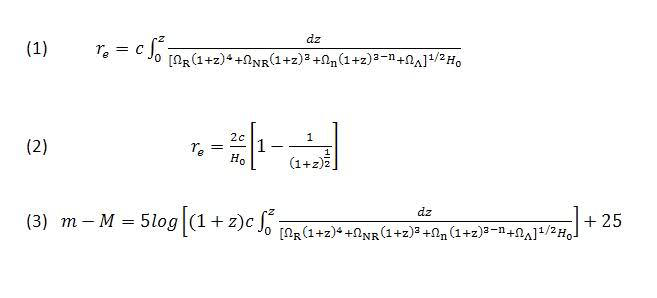# Density Parameters using Mathematica

## Homework Statement

hello, I have to solve a couple of equations on Mathematica for a project, and since they are not really working out, I wondered if you could help me out a bit.

I need to solve equation (1) (you can find the equations in the .doc attached) and plot the solution for a range of values of two omegas.
The omegas are related by the fact that the sum of all the omegas is always 1, so what we usually did in class was to assume was of them was 1 and the other 0, and we got something similar to equation (2).
Now I need to solve the equation with two non-zero gammas. So say, "NR" a "n".
The solution to the equation in my mathematica notebook doesn't seem right at all as it is lines long, and I'm supposed to write it down.
And the plot is quite unusual. It's discontinuos and stops at z=1 when it should be 0<z<10.

The second equation/plot are for equation (3). The plot looks reasonable, but it should look like the thick line in the image linked below
http://iopscience.iop.org/0004-637X/659/1/98/65868.fg6.html .
(The subscript "M" corresponds to our "NR")

I'm quite at a loss because it seemed a simple enough thing to do and the code looks alright, but I guess it's not.

Help! :)

#### Attachments

• dominations.nb
18.9 KB · Views: 190
• eqs-.doc
93 KB · Views: 144

diazona
Homework Helper
I can't speak for others, but I'd have a much easier time trying to help you out if I didn't have to open up a separate file just to see the problem. So you'll probably get quicker answers if you copy the relevant equations or whatever from the .doc file to your post.

Right, I need to solve equations (1) and (3) for two omegas only. That is, two of the omegas would be zero, whilst the other non-zero.And the sum of the omegas must always be 1.

What I've written so far in Mathematica is:
sol = NSolve[r2[z] == Integrate[(0.1*(1 + z)^3 + 0.9)^-0.5, z], r2[z]]

Plot[r2[z] /. sol, {z, 0, 10}, PlotRange -> {0, 10},
AxesLabel -> {z, Subscript[r, e]}]

sol1 = NSolve[
m[z] == 5 Log[(1 + z)*
NIntegrate[(0.27*(1 + z)^3 + 0.73)^-0.5, {z, 0, 10}] + 25 -
19.6], m[z]]

Plot[m[z] /. sol1, {z, 0, 10}, PlotRange -> {0, 20},
AxesLabel -> {z, m}]

diazona
Homework Helper
Are you supposed to solve for the omegas, or are you supposed to solve for $r_e$? Are the omegas constants or are they functions of $1 + z$? What about $r_e$, is that a function of z?

I'm supposed to solve for re for different values of the omegas.
so say 0.2, 0.8; 0.5, 0.5 and so on.
And the omegas are constant.

diazona
Homework Helper
In that case, I don't think there's any reason to use NSolve. You can just define r2 as a function of z,
Code:
r2[z_] := Integrate[..., {z,0,z}]